# Sin Cos Tan

Here we will learn about sin cos tan, including understanding what these three trigonometric functions are and their ratios, understanding when to use these functions and their inverse, and how to use them.

There are also sin cos tan worksheets based on Edexcel, AQA and OCR exam questions, along with further guidance on where to go next if you’re still stuck.

## What is sin cos tan?

Sin cos tan is a shortened description of the three trigonometric functions of sine, cosine, and tangent. These functions associate the ratio of two sides of a right-angled triangle with an angle.

To calculate using sin, cos and tan, we need to know their trigonometric ratios (remember that the ratio of two values is a division of these values). Consider the right-angled triangle below.

• \theta is the angle.
• The opposite side \textbf{(O)} is opposite to the angle.
• The adjacent side \textbf{(A)} is next to the angle.
• The hypotenuse \textbf{(H)} is the longest side (always the side facing the right-angle).

The three trigonometric ratios can be used to determine a missing side or a missing angle. We use the following trigonometric formulae.

Sine
Cosine
Tangent
\sin{\theta}=\frac{O}{H}
\cos{\theta}=\frac{A}{H}
\tan{\theta}=\frac{O}{A}

If we look at the letters only within the three triangles above, we get the abbreviation SOHCAHTOA where

• SOH is the association of the sine of an angle \text{(S)} with the opposite side \text{(O)} and the hypotenuse \text{(H)} of the triangle.
• CAH is the association of the cosine of an angle \text{(C)} with the adjacent side \text{(A)} and the hypotenuse \text{(H)} of the triangle.
• TOA is the association of the tangent of an angle \text{(T)} with the opposite side \text{(O)} and the adjacent side \text{(A)} of the triangle.

Step-by-step guide: SOHCAHTOA

We use one of the three trigonometric ratios of \textbf{sin θ}, \ \textbf{cos θ}, and \textbf{tan θ} to find one of the missing sides of a right angled triangle when we know another angle, apart from the right-angle.

We use the inverse functions of the three trigonometric ratios to determine a missing angle \theta (pronounced theta).

• The inverse sine function is written as \theta=\sin^{-1}\left(\frac{O}{H}\right).
• The inverse cosine function is written as \theta=\cos^{-1}\left(\frac{A}{H}\right).
• The inverse tangent function is written as \theta=\tan^{-1}\left(\frac{O}{A}\right).

Step-by-step guide: Trigonometric functions

### What is sin cos tan?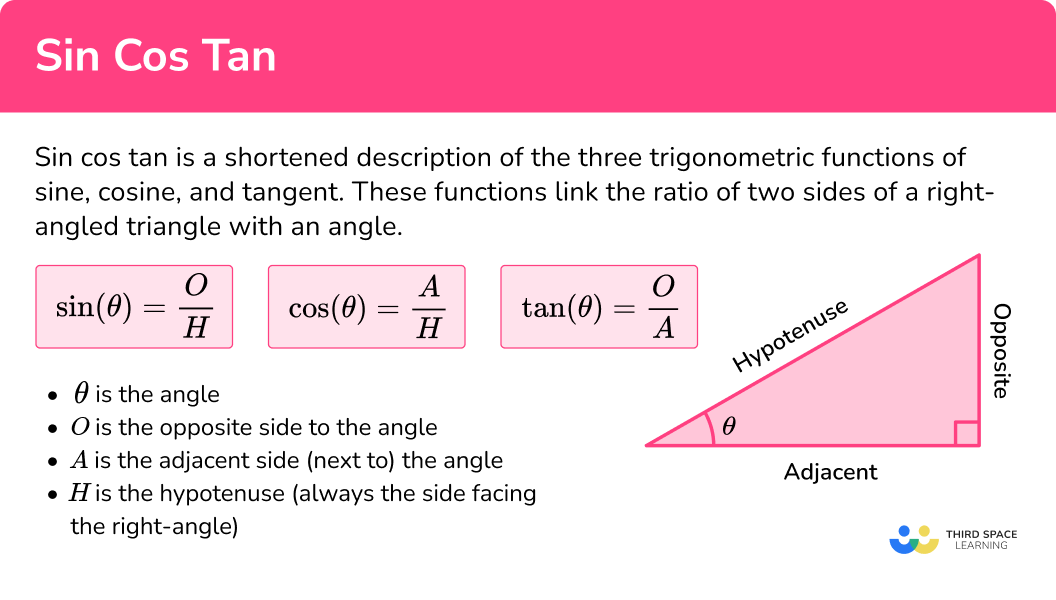## How to calculate using sin cos tan

In order to calculate using sin cos tan:

1. Label the sides of the right-angled triangle that we have information about in relation to the angle \textbf{θ} .
2. Choose the trig ratio we need.
3. Substitute the values from the triangle into the function.
4. Calculate the unknown side or angle, rearranging if necessary.

### Explain how to calculate using sin cos tan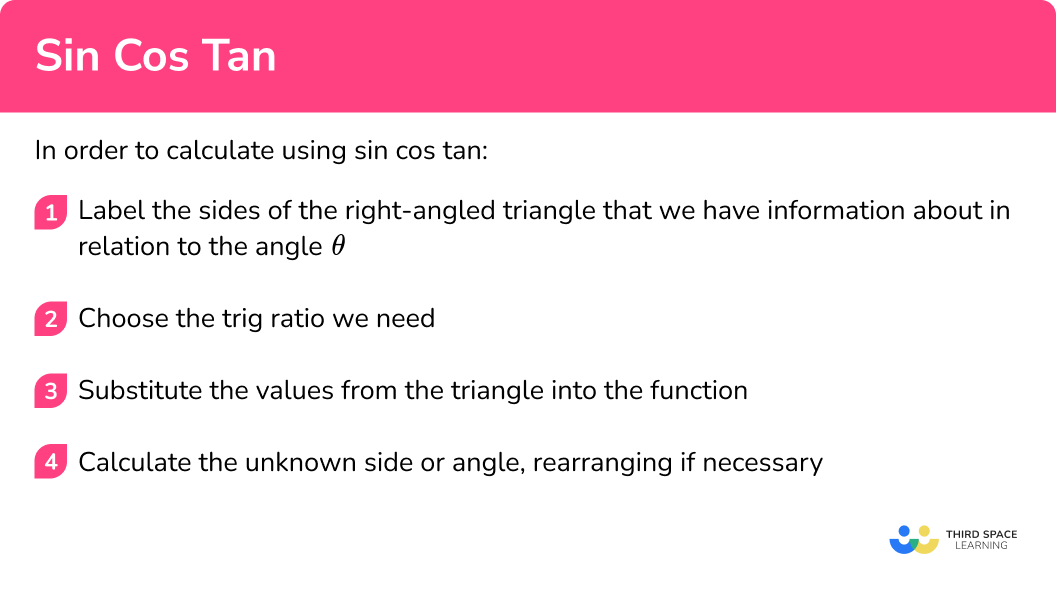## Sin cos tan examples

### Example 1: missing side (sine)

Determine the length of the hypotenuse for the triangle \text{ABC} below.

1. Label the sides of the right-angled triangle that we have information about in relation to the angle \textbf{θ} .

Labelling the sides in relation to the angle, we have,

2Choose the trig ratio we need.

We know that \ \theta=42^{\circ}, \ O=7 and H=x. Using SOHCAHTOA, we can use the sine ratio (SOH) to determine the missing length of the hypotenuse.

3Substitute the values from the triangle into the function.

Substituting these values into the trigonometry formula \sin \theta=\frac{O}{H}, we have

\sin{42}=\frac{7}{x}.

4Calculate the unknown side or angle, rearranging if necessary.

We need x to be the subject of the formula and so we first have to multiply both sides by x to get

x\times\sin{42}=7.

Dividing both sides of the equation by \sin 42, we get

x=\frac{7}{\sin{42}}=10.46133585…

The length of the hypotenuse x=10.46 \ cm\text{ (2dp).}

### Example 2: missing side (cosine)

Determine the length of the adjacent side for the triangle \text{PQR} below.

Label the sides of the right-angled triangle that we have information about in relation to the angle \textbf{θ} .

Choose the trig ratio we need.

Substitute the values from the triangle into the function.

Calculate the unknown side or angle, rearranging if necessary.

### Example 3: missing side (tangent)

Determine the length of the adjacent side for the triangle \text{EFG} below.

Label the sides of the right-angled triangle that we have information about in relation to the angle \textbf{θ} .

Choose the trig ratio we need.

Substitute the values from the triangle into the function.

Calculate the unknown side or angle, rearranging if necessary.

### Example 4: missing angle (sine)

Determine the size of the angle \theta for the triangle \text{ABC} below.

Label the sides of the right-angled triangle that we have information about in relation to the angle \textbf{θ} .

Choose the trig ratio we need.

Substitute the values from the triangle into the function.

Calculate the unknown side or angle, rearranging if necessary.

### Example 5: missing angle (cosine)

Determine the size of the angle \theta for the triangle \text{STU} below.

Label the sides of the right-angled triangle that we have information about in relation to the angle \textbf{θ} .

Choose the trig ratio we need.

Substitute the values from the triangle into the function.

Calculate the unknown side or angle, rearranging if necessary.

### Example 6: missing angle (tangent)

Determine the size of the angle \theta for the triangle \text{XYZ} below.

Label the sides of the right-angled triangle that we have information about in relation to the angle \textbf{θ} .

Choose the trig ratio we need.

Substitute the values from the triangle into the function.

Calculate the unknown side or angle, rearranging if necessary.

### Common misconceptions

• Make sure your calculator is set to degrees

All angles at GCSE are measured in degrees. Make sure your calculator screen is showing a small ‘D’ at the top before using it for trigonometry. If it is showing an ‘R’ it will measure the angle in radians which are not needed until A Level.

• Right triangle

Right-angled triangles are sometimes called right triangles.

• When to use SOHCAHTOA

We can use SOHCAHTOA to find a missing side of a right-angled triangle when we have another side and a given angle.

We can use SOHCAHTOA to find a missing angle of a right-angled triangle when we have two given sides.

If we have two sides and we want to find the third we can use the Pythagorean theorem a^{2}+b^{2}=c^{2}.

• Standard or inverse trig function

We use standard trig functions when we know the angle. We have to use the inverse trig functions when we don’t know the angle.

### Practice sin cos tan questions

1. Determine the length of the missing side of the right triangle below. Write your answer correct to 2 decimal places.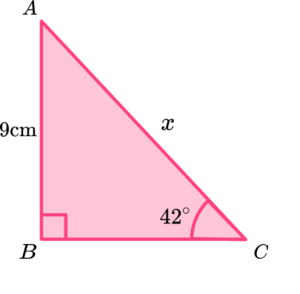4.67 \ cm6.02 \ cm12.11 \ cm13.45 \ cmFrom the angle \theta=42^{\circ} we know that O=9 and H=x so we use the sine ratio,

\sin\theta=\frac{O}{H}.

\sin{42}=\frac{9}{x}

x=\frac{9}{\sin{42}}=13.45028895…=13.45 \ cm\text{ (2dp)}

2. Determine the length of the missing side of the right triangle below. Write your answer correct to 2 decimal places.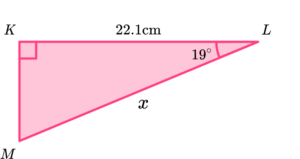20.90 \ cm23.37 \ cm64.18 \ cm67.88 \ cmFrom the angle \theta=19^{\circ} we know that A=22.1 and H=x so we use the cosine ratio,

\cos\theta=\frac{A}{H}.

\cos{19}=\frac{22.1}{x}

x=\frac{22.1}{\cos{19}}=23.37341705…=23.37 \ cm\text{ (2dp)}

3. Determine the length of the missing side of the right triangle below. Write your answer correct to 1 decimal place.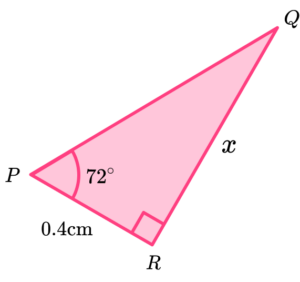28.8 \ cm1.2 \ cm0.1 \ cm7.7 \ cmFrom the angle \theta=72^{\circ} we know that O=x and A=0.4 so we use the tangent ratio,

\tan\theta=\frac{O}{A}.

\tan{72}=\frac{x}{0.4}

x=0.4\times\tan{72}=1.231073415…=1.2 \ cm\text{ (1dp)}

4. Determine the length of the missing angle \theta in the right triangle below.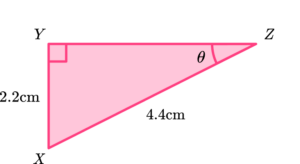26.57^{\circ}\text{ (2dp)}30^{\circ}60^{\circ}63.43^{\circ}\text{ (2dp)}From the angle \theta we know that O=2.2 and H=4.4. As we need to find the angle, we use the inverse sine ratio,

\theta=\sin^{-1}\left(\frac{O}{H}\right).

\theta=\sin^{-1}\left(\frac{2.2}{4.4}\right)=30^{\circ}

5. Determine the length of the missing angle \theta in the right triangle below. Write your answer to 1 decimal place.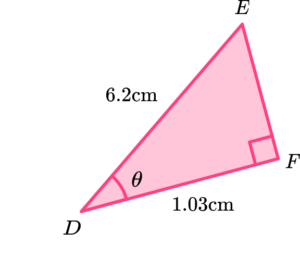9.4^{\circ}9.6^{\circ}48.4^{\circ}80.4^{\circ}From the angle \theta we know that A=1.03 and H=6.2. As we need to find the angle, we use the inverse cosine ratio,

\theta=\cos^{-1}\left(\frac{A}{H}\right).

\theta=\cos^{-1}\left(\frac{1.03}{6.2}\right)=80.4^{\circ} \ (1dp)

6. Determine the length of the missing angle \theta in the right triangle below. Write your answer to 2 decimal places.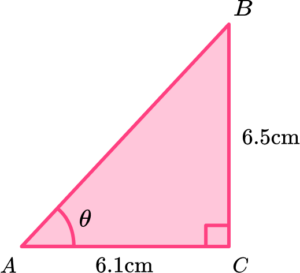43.18^{\circ}20.21^{\circ}69.79^{\circ}46.82^{\circ}From the angle \theta we know that O=6.5 and A=6.1. As we need to find the angle, we use the inverse tangent ratio,

\theta=\tan^{-1}\left(\frac{O}{A}\right).

\theta=\tan^{-1}\left(\frac{6.5}{6.1}\right)=46.81830296…=46.82^{\circ}\text{ (2dp)}

### Sin cos tan GCSE questions

1. Two planes take off from the same airport travelling at the same speed. Plane \text{A} flies East for 200 \ km. Plane \text{B} flies South for 150 \ km.

Determine the bearing of plane \text{A} from plane \text{B} at this time.

(3 marks)

\tan\theta=\frac{200}{150}

(1)

\theta=\tan^{-1}\left(\frac{200}{150}\right)

(1)

=53.1^{\circ}

(1)

Diagram: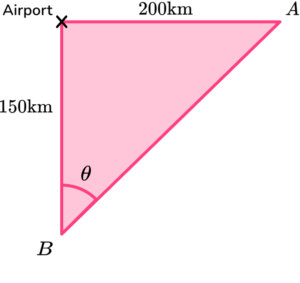2. Triangles \text{ABC} and \text{ACD} are joined along the side length \text{AC} such that \text{BCD} is a straight line. The length of \text{BC:BD} = 1:4.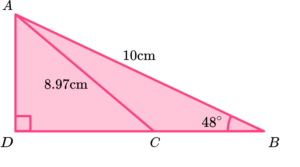(a) Determine the length of \text{CD} to 3 significant figures.

(5 marks)

(a)

BD=10\cos(48)=6.691…

(1)

CD=\frac{3}{4}\times{6.691…}

(1)

5.02 \ cm \text{ (3sf)}

(1)

Alternative method:

(1)

CD=\sqrt{8.97^2-7.4313…^2}

(1)

5.02 \ cm \text{ (3sf)}

(1)

(b)

\theta=\sin^{-1}\left(\frac{5.02}{8.97}\right)

(1)

\theta=34.03^{\circ}

(1)

Alternative method:

\theta=\sin^{-1}\left(\frac{5.02}{8.97}\right)

(1)

\theta=34.06^{\circ}

(1)

3. A man is standing 12.8 \ m from the wall of a block of flats, looking towards the top of the building at an angle of 76^{\circ}.

The man is 1.8 \ m tall. Determine the height of the block of flats, labelled x.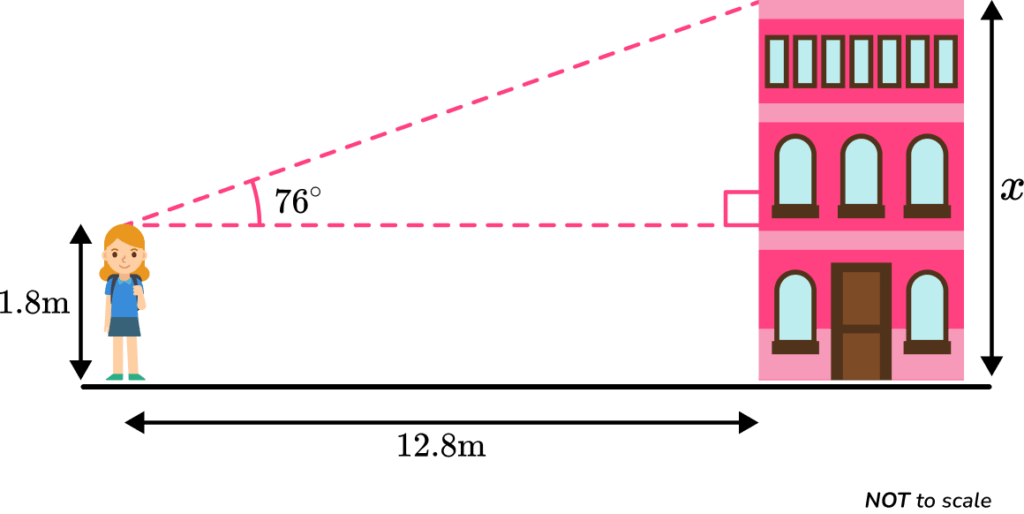(3 marks)

\tan(76)=\frac{O}{12.8}

(1)

51.337…+1.8

(1)

x=52.1 \ m \text{ (3sf)}

(1)

## Learning checklist

You have now learned how to:

• Apply trigonometric ratios to find angles and lengths in right-angled triangles

## Beyond GCSE

At A Level, we continue to develop understanding beyond the basic trigonometry functions of sine, cosine, and tangent. New functions that are studied include,

• The secant function (\sec \theta) where \frac{1}{\sin\theta}=\sec\theta.
• The cosecant function referred to as the cosec function (\csc \theta) where \frac{1}{\cos\theta}=\csc\theta.
• The cotangent function (\cot \theta) where \frac{1}{\tan\theta}=\cot\theta.

We study the derivatives of trig functions and are introduced to another unit of measure for angles called radians.

## Still stuck?

Prepare your KS4 students for maths GCSEs success with Third Space Learning. Weekly online one to one GCSE maths revision lessons delivered by expert maths tutors.

Find out more about our GCSE maths tuition programme.